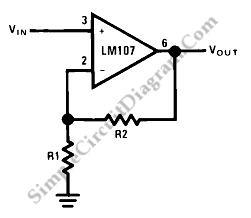# Op-Amp Application: Non-Inverting Amplifier

This is a high input impedance non-inverting circuit. This circuit provides a closed loop gain equal to (R1+R2)/R1. The close-loop 3 dB bandwidth of this circuit equal to the amplifier unity-gain frequency divided by the closed-loop gain. Here is the circuit :This circuit is different from an inverting circuit. The input impedance of this circuit is very high and equal to the differential input impedance multiplied by loop gain. In this circuit, the output is not inverted. The input impedance is not as important as the voltage and input current, if we uses the circuit in DC coupled applications.

When the input is allowed to float, the output  of amplifier will go into saturation. It should be noticed when the amplifier must be switched from source to source. [Source: National Semiconductor Application Note]